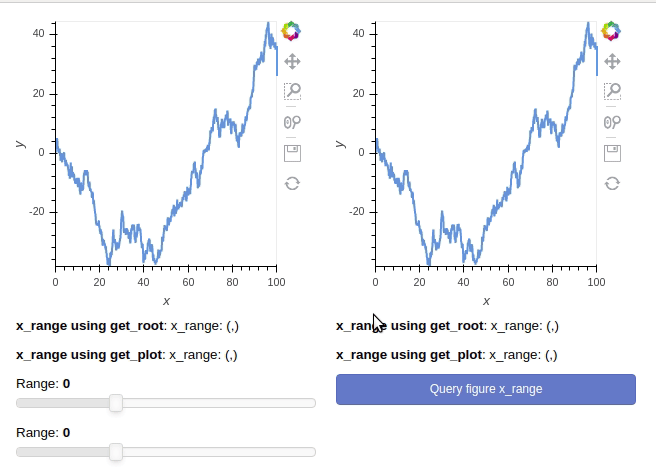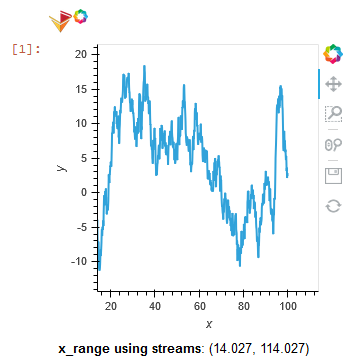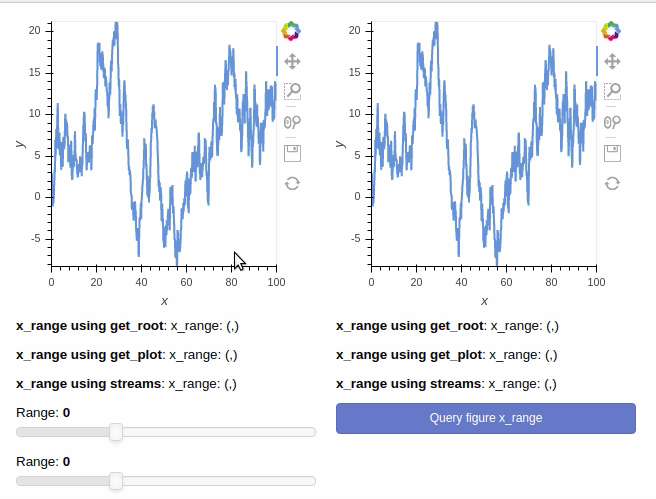# How to query currently visible holoviews plot range

I am trying to simply query the x_range of a holoviews plot. The issue I have is that unless the x_range limits are linked to a widget, the query always returns the range of the data, not the axis ranges.

I have tried querying the range using `get_root()` on the panel to return the bokeh doc, as well as using the holoviews `renderer.get_plot()` method and querying the handles dict.

The minimal example code simply creates 2 independent hv.Curve plots, and upon clicking the button, the x_range of the two plots is queried using the two methods defined above. The only case in which the correct current x_range is returned is when the plot x_range (the plot on the left) has a jslink with a panel widget. Otherwise the data range is always returned.

### Minimal example

``````# Example illustrating the output when the x_range of a hv.Curve plot is queried on a button click event

import holoviews as hv
import numpy as np
import pandas as pd
import panel as pn

hv.extension('bokeh')
renderer = hv.renderer('bokeh')

x_range_fmt = "({:.3f}, {:.3f})"

# Create dummy dataset
num_pts = 1000
df = pd.DataFrame({'x': np.linspace(0, 100, num_pts), 'y': np.cumsum(np.random.randn(num_pts))})

# Create scatterplots
hv_plots = [hv.Curve(df, 'x', 'y').opts(axiswise=True) for i in range(2)]

txt_xrange_doc = [pn.widgets.StaticText(name="x_range using get_root", value='x_range: (,)') for i in range(2)]
txt_xrange_renderer = [pn.widgets.StaticText(name="x_range using get_plot", value='x_range: (,)') for i in range(2)]

def query_x_range(event):
for i in range(2):
# Query x_range by using get_root on panel to obtain bokeh document
xrng_doc = panel.get_root().children[i].children.x_range
xrng_str = x_range_fmt.format(xrng_doc.start, xrng_doc.end)
txt_xrange_doc[i].value = xrng_str

# Query x_range by using holoviews renderer to get plot handles
xrng_renderer = renderer.get_plot(panel[i].object).handles['x_range']
xrng_str = x_range_fmt.format(xrng_renderer.start, xrng_renderer.end)
txt_xrange_renderer[i].value = xrng_str

update_button = pn.widgets.Button(name='Query figure x_range', button_type='primary')
update_button.on_click(query_x_range)

f1 = pn.widgets.FloatSlider(name="Range", start=-100, end=200, value=0.0)
f2 = pn.widgets.FloatSlider(name="Range", start=-100, end=200, value=0.0)

# Create panel
panel = pn.Row(pn.Column(hv_plots, txt_xrange_doc, txt_xrange_renderer, f1, f2),
pn.Column(hv_plots, txt_xrange_doc, txt_xrange_renderer, update_button))
panel.show()
``````

### Example code visualizationHi @mmorys,

How about using streams? More specifically `RangeX`.
http://holoviews.org/user_guide/Custom_Interactivity.html
http://holoviews.org/user_guide/Responding_to_Events.html

Here is a toy example that you may try to see if it fits with your needs:

``````import numpy as np
import pandas as pd
import holoviews as hv
import panel as pn

hv.extension('bokeh')
pn.extension()

num_pts = 1000
df = pd.DataFrame({'x': np.linspace(0, 100, num_pts), 'y': np.cumsum(np.random.randn(num_pts))})
hv_plot = hv.Curve(df, 'x', 'y')

rng = hv.streams.RangeX(source=hv_plot)

@pn.depends(rng.param.x_range)
def display_xrange(xr):
value = f"({xr:.3f}, {xr:.3f})" if xr else None
return pn.widgets.StaticText(name="x_range using streams", value=value, width=400)

app = pn.Column(hv_plot, display_xrange)
app
``````It’s likely the layout isn’t exactly what your after, I hope it’ll help you anywayThanks for the reply. This may be the cleanest way to go. I embedded your streams method in my example code to verify that the stream object can be queried using a button as well.

``````import holoviews as hv
import numpy as np
import pandas as pd
import panel as pn

hv.extension('bokeh')
renderer = hv.renderer('bokeh')

x_range_fmt = "({:.3f}, {:.3f})"

# Create dummy dataset
num_pts = 1000
df = pd.DataFrame({'x': np.linspace(0, 100, num_pts), 'y': np.cumsum(np.random.randn(num_pts))})

# Create scatterplots
hv_plots = [hv.Curve(df, 'x', 'y').opts(axiswise=True) for i in range(2)]

txt_xrange_doc = [pn.widgets.StaticText(name="x_range using get_root", value='x_range: (,)') for i in range(2)]
txt_xrange_renderer = [pn.widgets.StaticText(name="x_range using get_plot", value='x_range: (,)') for i in range(2)]
txt_xrange_stream = [pn.widgets.StaticText(name="x_range using streams", value='x_range: (,)') for i in range(2)]

# Create streams linked to x_range
rng = [hv.streams.RangeX(source=hv_plot) for hv_plot in hv_plots]

def query_x_range(event):
for i in range(2):
# Query x_range by using get_root on panel to obtain bokeh document
xrng_doc = panel.get_root().children[i].children.x_range
xrng_str = x_range_fmt.format(xrng_doc.start, xrng_doc.end)
txt_xrange_doc[i].value = xrng_str

# Query x_range by using holoviews renderer to get plot handles
xrng_renderer = renderer.get_plot(panel[i].object).handles['x_range']
xrng_str = x_range_fmt.format(xrng_renderer.start, xrng_renderer.end)
txt_xrange_renderer[i].value = xrng_str

# Query x_range stream object
xrng_stream = rng[i].x_range
xrng_str = x_range_fmt.format(xrng_stream, xrng_stream)
txt_xrange_stream[i].value = xrng_str

update_button = pn.widgets.Button(name='Query figure x_range', button_type='primary')
update_button.on_click(query_x_range)

f1 = pn.widgets.FloatSlider(name="Range", start=-100, end=200, value=0.0)
f2 = pn.widgets.FloatSlider(name="Range", start=-100, end=200, value=0.0)